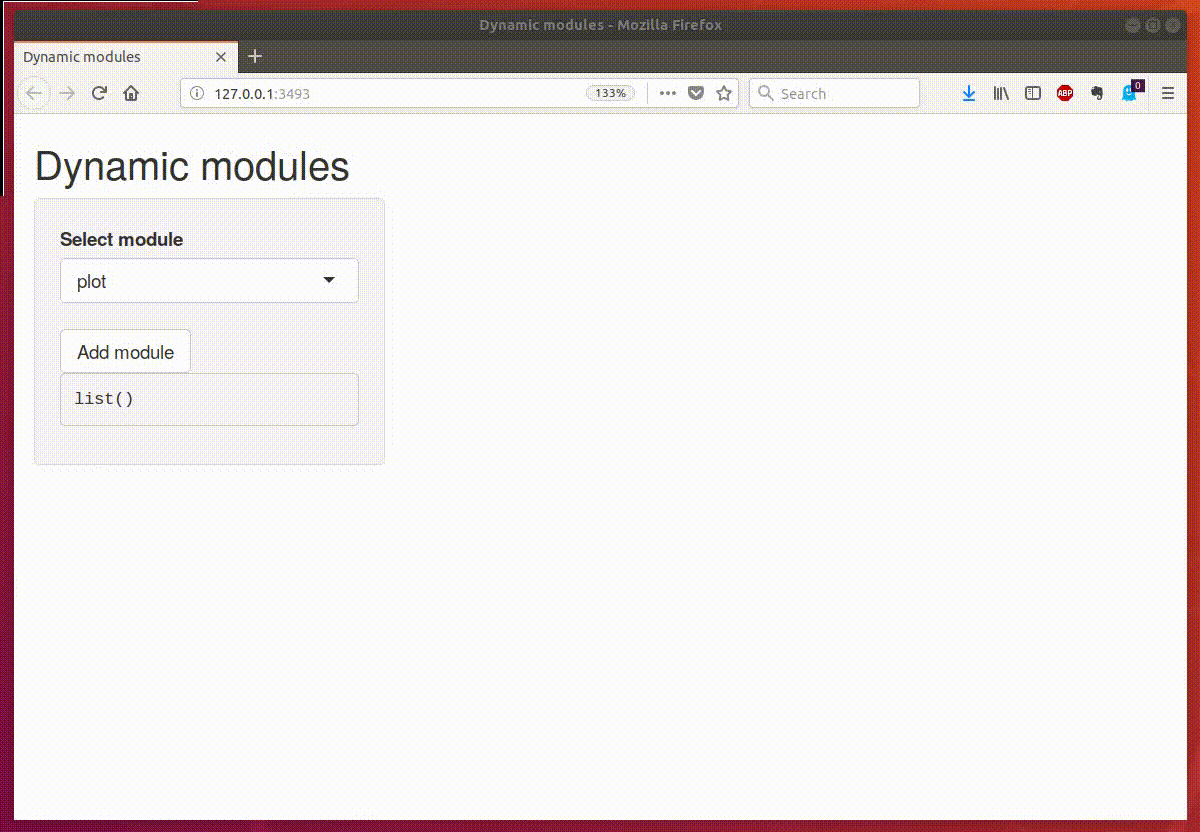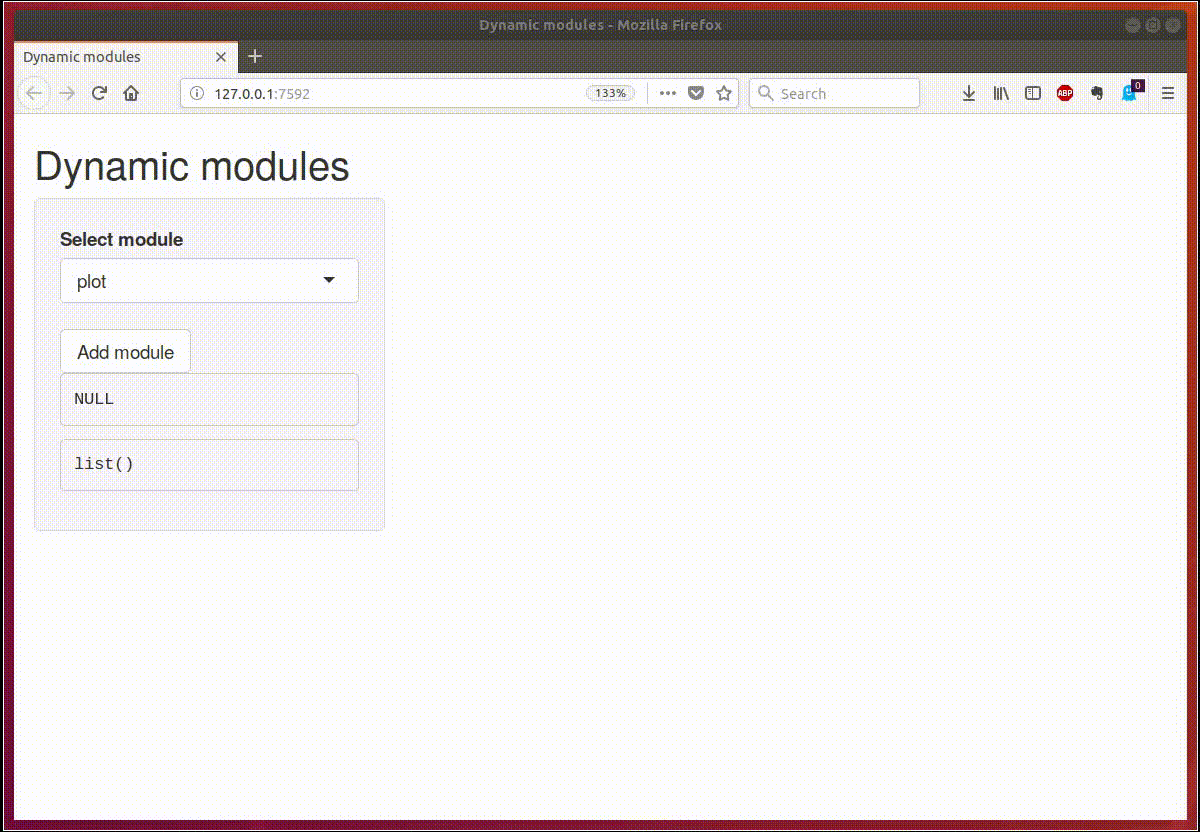In the previous post about shiny modules, I described how to dynamically add new modules, or even select a module type from a few possibilities. The main problem was that the new module is added inside an observer function, so we cannot directly get the returned value of the new modules.

However, we can solve this problem quite easily using reactiveValues. We just add a parameter to the module’s server function in which we will send the reactive value used to communicate between the main application and the module.

In the example below, we use a state reactiveValues object to send the message from the module to the main application (this approach can also be used to communicate between modules). Note that the state is initialized as an empty object, and new elements are added inside the modules, but the renderPrint works as expected, it shows the values from all existing modules.

library(shiny)
##### Plot module

modulePlotUI <- function(id) {
ns <- NS(id)
fluidRow(
column(6, sliderInput(ns("n"), label = "N:", min = 10, max = 100, value = 50)),
column(6, plotOutput(ns("plot"), height = "200px"))
)
}

modulePlot <- function(input, output, session, state) {

output$plot <- renderPlot({ # send all values from the module as a element of the state object state[[session$ns("id")]] <- list(N = input$n, type = "plot") plot(rnorm(input$n), rnorm(input$n)) }) } ##### Text module moduleTextUI <- function(id) { ns <- NS(id) fluidRow( column(6, sliderInput(ns("n"), label = "N:", min = 10, max = 20, value = 10)), column(6, verbatimTextOutput(ns("text"))) ) } moduleText <- function(input, output, session, state) { output$text <- renderPrint({
state[[session$ns("id")]] <- list(N = input$n, type = "text") # save state to the state object
sample(LETTERS, input$n, replace = TRUE) }) } ui <- fluidPage( titlePanel("Dynamic modules"), sidebarLayout( sidebarPanel( selectInput( inputId = "type", label = "Select module", choices = c("plot", "text")), actionButton("Add", label = "Add module"), verbatimTextOutput("ModuleText"), verbatimTextOutput("AllModulesText") ), mainPanel( div(id = "addPlaceholder") ) ) ) server <- function(input, output) { state <- reactiveValues() output$AllModulesText <- renderPrint(reactiveValuesToList(state))

idCounter <- reactiveVal(value = 0)
observeEvent(input$Add, { id <- idCounter() selected <- if(input$type == "plot") {
list(ui = modulePlotUI, server = modulePlot)
} else if(input$type == "text") { list(ui = moduleTextUI, server = moduleText) } insertUI( selector = "#addPlaceholder", where = "beforeBegin", ui = selected$ui(paste0("id",id)))

callModule(
module = selected$server, id = paste0("id",id), state = state) idCounter(id + 1) }) } shinyApp(ui = ui, server = server) And the GIF of the working machinery (note the text output on the left side changes when the value on module’s slider moves).There’s one more thing. It might be easier to reason about the application when you use two reactiveValues. One for keeping the state of each module, and the second for sending specific actions to the other parts of the application. This leads us to the second version of the example app in which there’s one output which shows the content of the last clicked module. library(shiny) ##### Plot module modulePlotUI <- function(id) { ns <- NS(id) fluidRow( column(6, actionButton(ns("Action"), label = "Show me"), sliderInput(ns("n"), label = "N:", min = 10, max = 100, value = 50)), column(6, plotOutput(ns("plot"), height = "200px")) ) } modulePlot <- function(input, output, session, state, action) { output$plot <- renderPlot({
# send all values from the module as a element of the state object
state[[session$ns("id")]] <- list(N = input$n, type = "plot")
plot(rnorm(input$n), rnorm(input$n))
})

observeEvent(input$Action, { action$action <- state[[session$ns("id")]] }) } ##### Text module moduleTextUI <- function(id) { ns <- NS(id) fluidRow( column(6, actionButton(ns("Action"), label = "Show me"), sliderInput(ns("n"), label = "N:", min = 10, max = 20, value = 10)), column(6, verbatimTextOutput(ns("text"))) ) } moduleText <- function(input, output, session, state, action) { output$text <- renderPrint({
state[[session$ns("id")]] <- list(N = input$n, type = "text")
sample(LETTERS, input$n, replace = TRUE) }) observeEvent(input$Action, {
action$action <- state[[session$ns("id")]]
})

}

ui <- fluidPage(
titlePanel("Dynamic modules"),
sidebarLayout(
sidebarPanel(
selectInput(
inputId = "type",
label = "Select module",
choices = c("plot", "text")),
verbatimTextOutput("ModuleText"),
verbatimTextOutput("AllModulesText")
),
mainPanel(
)
)
)

server <- function(input, output) {
state <- reactiveValues()
action <- reactiveValues(action = NULL)
output$ModuleText <- renderPrint(action$action)
output$AllModulesText <- renderPrint(reactiveValuesToList(state)) idCounter <- reactiveVal(value = 0) observeEvent(input$Add, {
id <- idCounter()

selected <- if(input$type == "plot") { list(ui = modulePlotUI, server = modulePlot) } else if(input$type == "text") {
list(ui = moduleTextUI, server = moduleText)
}

insertUI(
where = "beforeBegin",
ui = selected$ui(paste0("id",id))) callModule( module = selected$server,
id = paste0("id",id),
state = state,
action = action)
idCounter(id + 1)
})

}

shinyApp(ui = ui, server = server)

And the GIF:If you have any question related to the article, send me a message on Twitter, LinkedIn, Github (https://github.com/zzawadz/blog/issues) or email.

The source code for both applications are here: